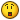## Recommended Posts

Alright what i have is, two combo boxes and an INI. This ini contains ZONES which get put into the first combo box. I have gotten the ZONES into the first combo, but what i need some help with is:

I need it so when you select a ZONE in the first combo box, it finds every MAP thats in that zone and puts it in the combo box, then if you switch ZONE it does it again. I also need to assain each of the MAPs a few variables such as NODENUM, NAME, ID and Node0 through Node[NODENUM - 1]=****. Then i need to add the NAME of each of those found MAPs into the second combo box. How would i go about doing this? Would i need to create a huge array with multiple arrays inside of it or something?

Heres an example of what the INI looks like:

```[zone]
Zone0=WaterCity
Zone1=TreeCity
Zone2=HillCity
Zone3=NewHillCity
Zone4=SandCity
Zone5=BanditCave
Zone6=RichPlain
Zone7=ForbiddenCity
Zone8=SnowCity
Zone9=SeaShore
Zone10=OceanCity
Zone11=HuaMountain
Zone12=WuDang
Zone13=Shaolin
Zone14=HeroicTomb
Zone15=SeniorGodCave
Zone16=Hell
Zone17=SkyCity
Zone18=NewSkyCity
Zone19=Unknown
Zone20=DevilCave

;~~~~~~~~~~~~~~~~~~~~~~~~~~~~~~~~~

[map0]
Id=100000
Zone=0
Name=Nurse
NodeNum=1
Node0=24,17,100001
[map1]
Id=100001
Zone=0
Name=WaterCity
NodeNum=13
Node0=79,66,100032
Node1=79,72,100000
Node2=102,81,100033
Node3=100,74,100034
Node4=62,79,100031
Node5=81,23,100014
Node6=4,63,100011
Node7=112,75,100030
Node8=61,84,100091
Node9=-1,1,100089
Node10=-1,25,100002
Node11=-1,26,100003
Node12=-1,27,100009

[map2]
Id=100002
Zone=2
Name=HillCity
NodeNum=10
Node0=107,95,100021
Node1=31,26,100058
Node2=84,75,100057
Node3=62,29,100056
Node4=52,85,100060
Node5=19,42,100059
Node6=22,37,100055
Node7=-1,22,100001
Node8=-1,23,100003
Node9=-1,24,100009```

Can someone please help me out? ThanksEdited by jackyyll

##### Share on other sites

How do you know which zone goes with which map? The reason I ask is there are less maps then there are zones it appears.

Common sense plays a role in the basics of understanding AutoIt... If you're lacking in that, do us all a favor, and step away from the computer.

##### Share on other sites

Yeah i know thats just an example because the actual file is huge##### Share on other sites

Yeah i know thats just an example because the actual file is hugeOk, I understand, but in order to help you ... or show you how to put these into a UDF or an Array to pull properly... so that you aren't asking a ton more questions on how to get it to work, you should provide specifics on what goes with what... kind of like a map, steps to what you want.

Example:

Read Zone1 >> Go To Map 1 >> Pull Info >> Match to Map 4 >> Compare Diff >> Load Diff to Combo Box (not a correct example I'm sure, but demonstrating on how to make the answers you get more efficient).

Common sense plays a role in the basics of understanding AutoIt... If you're lacking in that, do us all a favor, and step away from the computer.

##### Share on other sites

Well what im trying to do is fill the second combo box with the maps that are in the selected zone.. So say Zone0 is selected, i want it to fill the combo box with all the map names that say zone=0. and i want each of those maps in the second combo to hold data.. like how many nodes there are the id of the map . Also i want it to hold the node0=**,**,****** each of the things seperated by commas needs to be kept seperately as values so if node0=12,34,100000 i need \$var1 = 12 and var2=34 and var3=100000. Not sure how possible this is, but i guess thats what i need to gather that information so that i can move maps and such.

##### Share on other sites

anyone know how to do this? :/

##### Share on other sites

```For \$i = 0 To 100
If \$read = "default" Then ExitLoop
Next```

you can do an if-condition, too, which asks if the read is 0... if its true you can put it into a list view or combo f.e.

Edited by Lord_Doominik

## Create an account

Register a new account

• ### Recently Browsing   0 members

×

• Wiki

• Back

• #### Beta

• Git
• FAQ
• Our Picks
×
• Create New...